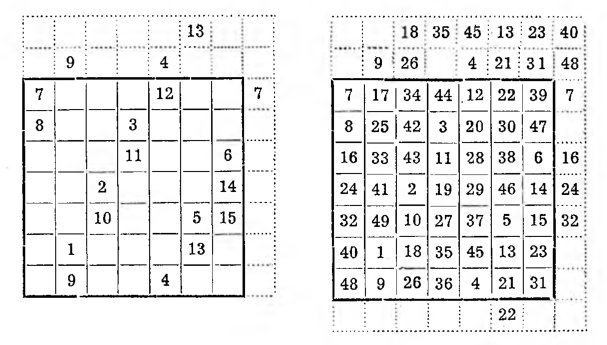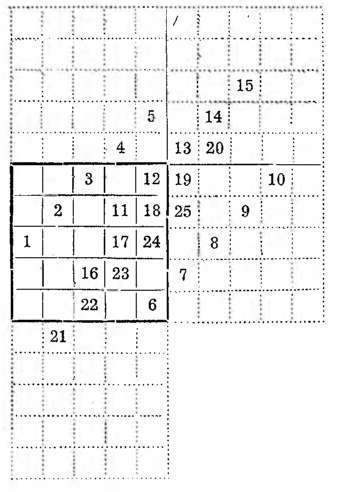# Construction of odd magic squares part 2IV. La Louber'a Method. We will show it on the square of the fifth row. Instead of the pyramids, four other squares of the same size are added to the main square, thus the figure shown opposite is obtained.

In the middle field of the left column, enter 1 and going diagonally to the top right, enter the numbers 2, 3, 4, 5.

After entering the top five, we stop.

Numbers 4 and 5 they went beyond the square. When we move each of them by 5 boxes down, they will then be inside the square (see the finished square in the next figure).

Under this new position of the number 5 we type 6 and again going right towards. write at the top 7, 8, 9, 10, After entering the second five, we see, that numbers 7, 8, 9, 10 they went beyond the square; we square them by shifting by 5 fields to the left. Under the new position of the number 10 we type 11 and next 12, 13, 14, 15.

To put numbers in a square 13, 14, 15, you will have to move them by 5 fields down and o 5 fields to the left. We do this until the end.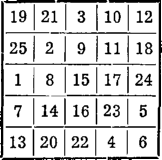When we come to the last word of progress, that is, as for now, into 25, then all numbers standing in additional squares are transferred to the corresponding area of ​​the main square and a magic square different from the previous one is obtained, obtained by the Bachet method. Under the field 25 there will be a box 1.

V. A variation on the previous method. Inspired by the thought of La L o u b e r e, we present a variant of his method which gives symmetrical magic squares of fifth order. Well, we write out on squared paper the sequence of numbers arranged in this way;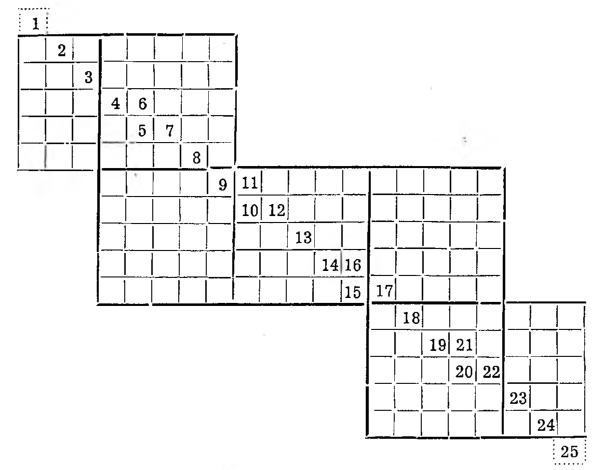After entering all the numbers into the squares, draw such a square with thicker lines, so that the numbers of the primary group lie on its diagonal: 11, 12, 13, 14, 15, and then - adjusting to this square - we use thicker lines to draw other squares of the fifth row or their parts.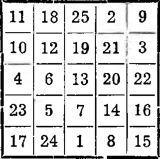Now it will not be difficult to move all the numbers inside the main square and get the magic square you are looking for. It is, as easy to tell, symmetrical magic square.

Symmetrical squares have the interesting property of transforming into squares of a different type in an original way. You can move the second row of numbers one square to the right, then the third row to the right, and so on, then move the whole triangle of the numbers to the empty boxes on the left, as shown in the diagram: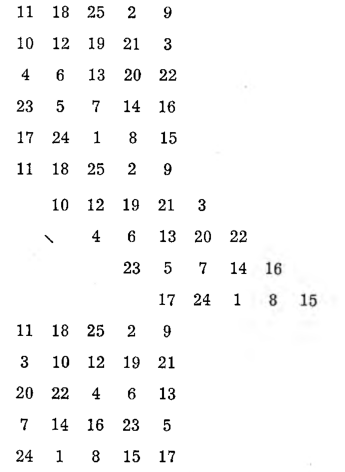We got the magic square again, but already asymmetrical.

WE. The method of jumping a chess horse, very original, and at the same time easy and interesting. This time we will take the square of the seventh row as an example, that is, forty-nine-field. We place one in any field, above her 2, 3 and so on, enter in the fields, on which the chess horse would have jumped. The four will go beyond the square, so you need to move it to an analogous square inside the square and continue jumping from it. When we get to seven, and then to further multiples 7, that is, to 14, 21, . . ., consecutive, tj. 8, 15, 22, . . . we sign in the field directly below and from it again we place further numbers with jumps of a tailstock, until we get to 49.